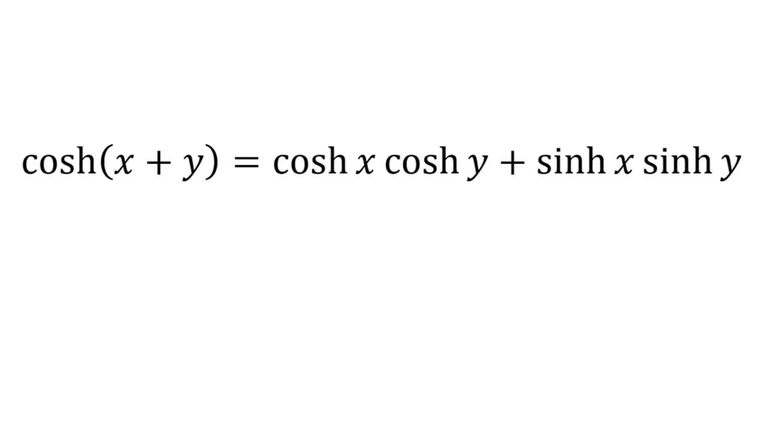# Hyperbolic Trigonometric Identity: cosh(x+y)

in Threespeak2 months agoIn this video I go prove the hyperbolic trigonometric identity cosh(x+y) = cosh x cosh y + sinh x + sinh y. I had proved same identity several years back in my earlier video, but that proof simply involved back-calculating that the identity was correct. In this video though, I show how to go about proving the identity without knowing the final result. I show this by illustrating that the cosh(x + y) involves a multiplication of exponents, which can also result by multiplying cosh and sinh functions together in several variations. Thus I show how to reverse a foil expansion process and ultimately obtain cosh(x+y) in terms of cosh x, cosh y, sinh x, and sinh y. This is a very interesting proof video in that I show that we need to think ahead at what we ultimately want before we begin attempting to derive an identity; so make sure to watch this video!

Download the notes in my video: https://1drv.ms/b/s!As32ynv0LoaIhvtVnszc8jhu0oC3-Q

View video notes on the Hive blockchain: https://peakd.com/mathematics/@mes/video-notes-hyperbolic-trigonometric-identity-cosh-x-y

Related Videos:

Hyperbolic Functions - tanh(x), sinh(x), cosh(x) - Introduction: http://youtu.be/EmJKuQBEdlc
Hyperbolic Trigonometry Identity Proof: sinh(x+y) = sinh(x)cosh(y) + cosh(x)sinh(y): http://youtu.be/hrOId-Yp2rk
Hyperbolic Trigonometry Identity Proof: cosh(x+y) = cosh(x)cosh(y) + sinh(x)sinh(y): http://youtu.be/UEHQ8SSqDXc .

SUBSCRIBE via EMAIL: https://mes.fm/subscribe

DONATE! ʕ •ᴥ•ʔ https://mes.fm/donate

Like, Subscribe, Favorite, and Comment Below!

Follow us on:

MES Truth: https://mes.fm/truth
Official Website: https://MES.fm
Hive: https://peakd.com/@mes
Gab: https://gab.ai/matheasysolutions
Minds: https://minds.com/matheasysolutions
Twitter: https://twitter.com/MathEasySolns
Facebook: https://fb.com/MathEasySolutions
LinkedIn: https://mes.fm/linkedin
Pinterest: https://pinterest.com/MathEasySolns
Instagram: https://instagram.com/MathEasySolutions
Email me: [email protected]

Free Calculators: https://mes.fm/calculators

BMI Calculator: https://bmicalculator.mes.fm
Grade Calculator: https://gradecalculator.mes.fm
Mortgage Calculator: https://mortgagecalculator.mes.fm
Percentage Calculator: https://percentagecalculator.mes.fm

Free Online Tools: https://mes.fm/tools

iPhone and Android Apps: https://mes.fm/mobile-apps

▶️ 3Speak